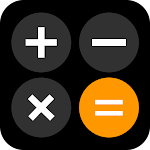ID: com.calculator.ct

## The description of iOS 16 Calculator: iCalculator

New Calculator with all-purpose ios Calculator featuring all scientific functions to solve complex mathematical problems just like an iOS 16 calculator 🚀🚀🚀

iOS 16 calculator has an appealing appearance and is a free Android calculator. You will most likely never need another calculator app after this one. This basic calculator offers an excellent user experience and strong features. The full ios calculator helps you save significant time and effort.

Calculator App has numerous well-designed calculator themes available on this calculator pro app, and there are math calculator customization choices for the display format. Large buttons and lettering of this Calculator pro makes it impossible for you to misjudge what you are touching or seeing in this calculator pro.

iOS calculator also provides accommodated history. You can retrieve any extensive computation in this Basic Calculator that you have conducted whenever you want because it is recorded in a database of icalculator. This fast calculator helps you to remember each calculation that you do while using our calculator app.

🚀BASIC AND ADVANCED IOS 16 CALCULATOR🚀

iOS 16 calculator is a simple and easy calculator with history. This basic calculator is a unique math helper app that is designed to solve different kinds of mathematical problems from simple to complex. The Scientific calculator is a free math solver app that comes in two modes, portrait, and landscape. The portrait mode presents you with basic mathematical functions. This calculator app for android includes addition, subtraction, multiplication, and division. The landscape mode of this amazing app features hyperbolic, logarithmic, and trigonometry functions. Our calculator app is your solution to all mathematical problems.

🚀GET MATH HELP WITH TWO CALCULATOR MODES🚀

Math Helper calculator offers degree and radiant features. In this ios calculator, you can easily change the calculator mode from degree to radiant and vice versa depending on the equation. Every mathematical icalculator has you covered. Just download this minimalistic calculator and enjoy it. However, this android calculator has a multifunctional calculator. The average person hardly ever uses multi-digit calculators, scientific calculators, or other calculators with sophisticated functionality.

🚀ALGEBRA CALCULATOR IS SUITABLE FOR ALL 🚀

Math Calculator is a unique Calculator x app that is suitable for every age. This calculator app has two modes that allows users to operate simple as well as advanced functions. So, whether you are educated or not, this Calculator App is a solution for you. This math calculator contains a (%) button, so you can quickly calculate discounts, tipping, or anything else that involves percentages. You can use the ios calculator every day for tasks like homework and shopping as well as for calculating bills, payments, and receipts. It is completely free to use and a very basic calculator.

🚀CALCULATOR PRO WITH FUN THEMES🚀

iCalculator was developed keeping in mind the strain of constant light. Therefore, the calculator app is designed in the dark mode so that you can keep using it for longer hours. This Math Solver comes with a catchy combination of orange to make the experience fun and interesting for you. You will love using this Calculator app because of the classy and attractive color scheme, which won't strain your eyes. In this calculator, you may do mathematical operations including trigonometric, logarithmic, and exponential functions.

🚀iOS 16 CALCULATOR KEY FEATURES🚀

🚀Calculate Basic calculations with Algebra Calculator
🚀Math Calculator checks errors automatically
🚀Large input/output display in Math Calculator